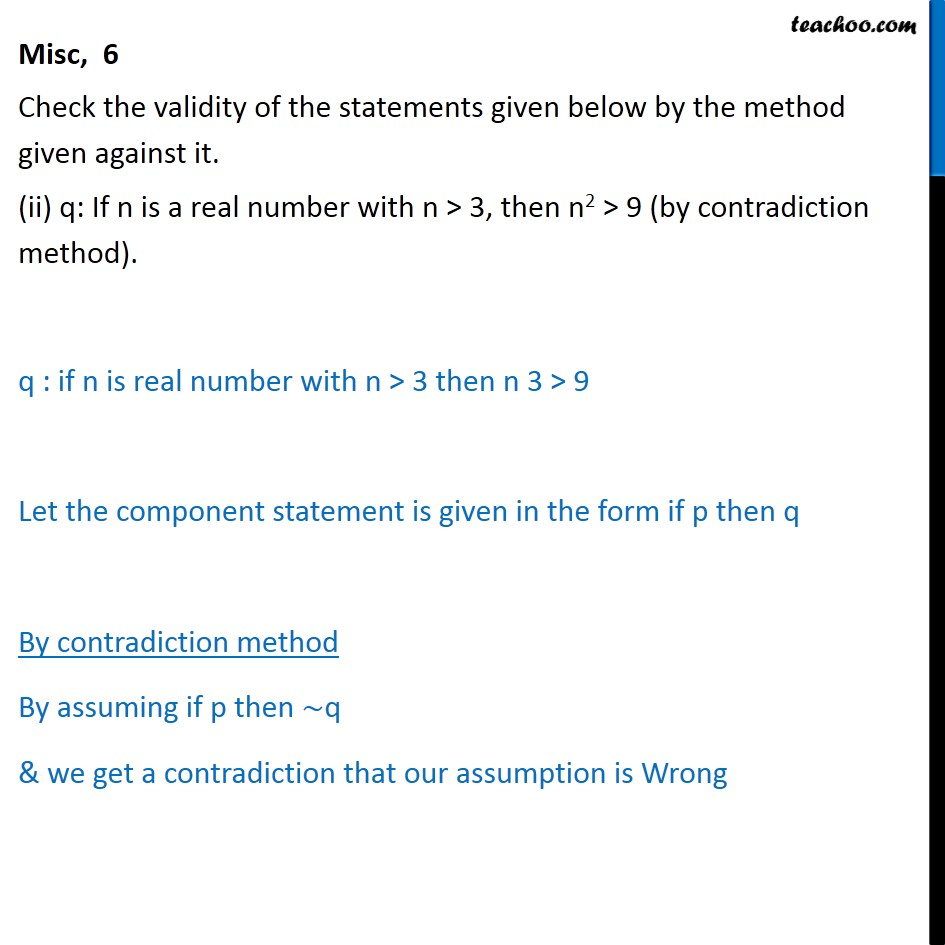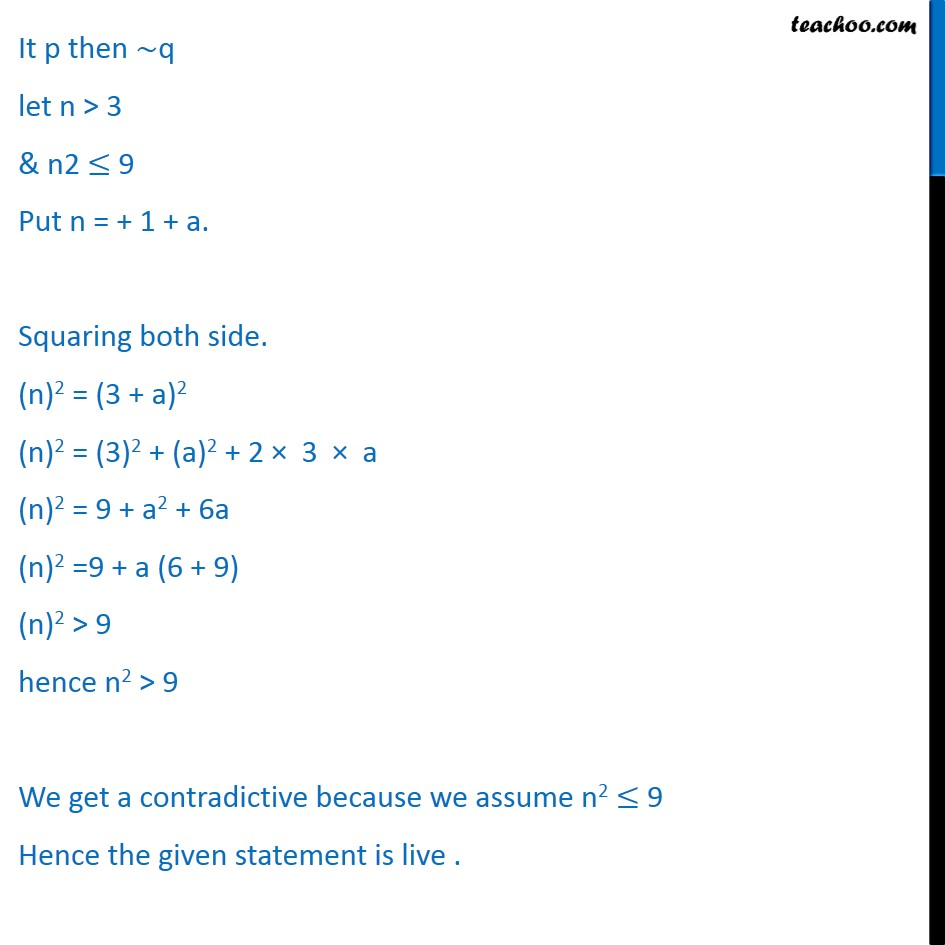Miscellaneous

Chapter 14 Class 11 Mathematical Reasoning
Serial order wiseGet live Maths 1-on-1 Classs - Class 6 to 12

### Transcript

Misc, 6 Check the validity of the statements given below by the method given against it. (ii) q: If n is a real number with n > 3, then n2 > 9 (by contradiction method). q : if n is real number with n > 3 then n 3 > 9 Let the component statement is given in the form if p then q By contradiction method By assuming if p then q & we get a contradiction that our assumption is Wrong It p then q let n > 3 & n2 9 Put n = + 1 + a. Squaring both side. (n)2 = (3 + a)2 (n)2 = (3)2 + (a)2 + 2 3 a (n)2 = 9 + a2 + 6a (n)2 =9 + a (6 + 9) (n)2 > 9 hence n2 > 9 We get a contradictive because we assume n2 9 Hence the given statement is live .